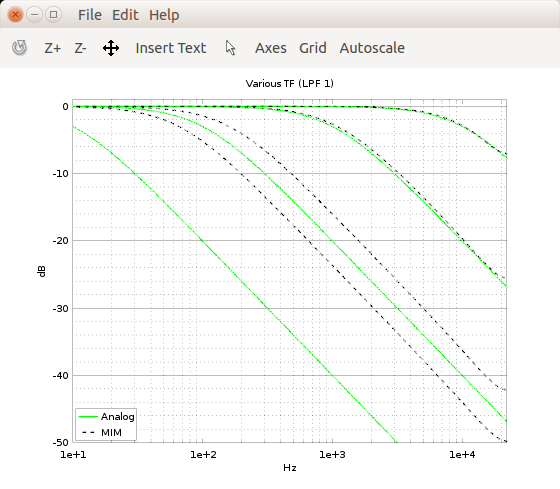## What is the reason for this 'linear error'Started by 6 years ago5 replieslatest reply 6 years ago131 views

Hi!

Here's MIM (Magnitude Invariance Method) paper (press the "View PDF" to get the paper/matlab code in hands) and here's a plot showing the issue I'm trying to solve:Magnitude responses for one pole LP filter at few frequency locations.

As plot shows, there's some issue in MIM's function c2dn() implementation, which 'moves' the fc point especially at low fc values. IIRC, same type of issue happens when changing the fs.

Analog prototype LPF is calculated as:

w0 = 2*pi*fc;
Analogb = 1;
Analoga = [1 w0];
Analog = tf(Analogb, Analoga);
Analog = Analog/dcgain(Analog);

which is then given as an input for to calculate the MIM filter:

fs=44100;
mimpim = 'mim';
samplingtime = 1/fs;
numofsamples = 2048;
[Dz1] = c2dn(Analog, samplingtime, mimpim, 1, numofsamples);
%[a2, b2, T] = tfdata(Dz1);   %coeffs

where c2dn() is the function from MIM paper.

Plotting:

fs2 = fs/2;
nf = logspace(0, 5, fs2);
[mag, pha] = bode(Analog,2*pi*nf);
semilogx(nf, 20*log10(abs(mag)), 'color', 'g', 'linewidth', 1.5);
axis([10 fs2 -50 1]);
hold on;[mag, pha] = bode(Dz1,2*pi*nf);
semilogx(nf, 20*log10(abs(mag)), 'color', 'k', 'linewidth', 1.5, 'linestyle', '--');grid on;
title('Various TF (LPF 1)');
legend('Analog', 'MIM','location', 'southwest');
xlabel('Hz');ylabel('dB');

So far I found out that the fc error is quite linear between certain points (I picked -3dB points for few fc and then calculated the trend f(x) (R² was 0.99989...)) so, one could probably find the source for "error" by looking the matlab codes for MIM function c2dn().

Here's plot showing the effect of my correction f(x):Correction formula f(x) = 0.9704384746x - 51.6991952722 works well for fc in range 60Hz-Nyqvist (fs=44.1kHz). (Note: correction for fc=10Hz showen in plot  is done using another formula so you can omit it as a result of this f(x)).

Any suggestions regarding the source for this "error"?

[ - ]Hi!

I think it would be convenient if you give explicit examples of expectation vs outcome and also put the related formulas and/or code snippets so the reader doesn't have to waste time studying the paper and code in order to help you. Because just looking at the plot I don't understand what is "MIM" and where the "linear error" is that you are trying to explain (and/or fix or avoid).

Cheers

[ - ]Looks like the 1st image showing the error was not present anymore? I added it and also added some Octave code which I used in making the LP filters and 1st plot.

Matlab code for function c2dn() is copyrighted so I can't list it here.

[ - ]One more note: Decreasing the value of numofsamples parameter improves response at lower fc (and vice versa) ?[ - ]I quess the functionality behind the issue can be found from cepstral processing block ... there's a line with remark: "% minimum phase sequence r^mn" which changes h data by taking lmn data (
matrix lmn = [0.5 1 1 1 1 1 1 1 1 1 1 1 1 1 1 1 1 1 1
1 1 1 1 1 1 1 1 1 1 1 1 1 1 1 1 1 1 1 1
1 1 1 1 1 1 1 1 1 1 1 1 1 1 1 1 1 1 1 1
1 1 1 1 1 0 0 0 0 0 0 0 0 0 0 0 0 0 0 0
0 0 0 0 0 0 0 0 0 0 0 0 0 0 0 0 0 0 0 0
0 0 0 0 0 0 0 0 0 0 0 0 0 0 0 0 0 0 0 0
0 0 0 0 0 0 0 0 0]

(above matrix actual when numofsamples = 128)) from "homomorphic filtering" block and calculates new values for h:

h = h.*lmn';

When this line is in comments magnitude plots looks good (if the numofsamples parameter has suitable (big) value):Problem is that commenting the line effects directly to the aliasing issue this type of filter hasAny thoughts if this (r^mn thingy) can be improved somehow?
[ - ]